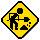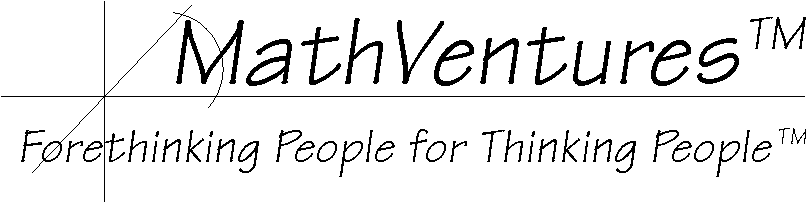Hard hat area, construction in progressLogic  the Art of Reasoning

Mathematics   the Art of Studying Patterns Using Logic

Here Is an ExceptionIn other word, is

a + b = b + a

always true?

Here is an exception:
How about the graphic example below. In it, a and b are not simple numbers. Each of them is a 90 degree rotation and the addition operation is the result of two such rotations, the second of which must not be in the same plane as the first.As you see, in the graphic example above, the result of adding up these two 90 degree turns (rotations) is not the same. In other words,

a + b != b + a.
(if you are not familiar with th symbol  !=, it means not equal.)